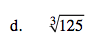### Home > AC > Chapter 14 > Lesson 14.3.1.5 > Problem3-68

3-68.
1. The solution to the equation x 3 =64 is called the cube root of 64. The idea is similar to the idea of a square root, except that the value must be cubed (multiplied by itself three times) to become 64. One way to write the cube root of 64 is using the notation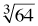. Use this information to evaluate each of the following expressions. Homework Help ✎

1.2.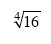3.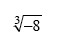4.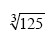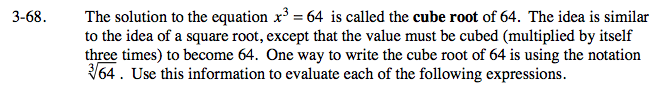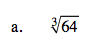4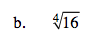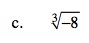−2